## 2-3 Search Trees

Recall that binary search trees have fast average search and insert comparison costs. However, as the connection between binary search trees and quicksort revealed, the cost for any given binary search tree depends highly on its shape, which in turn depends on the order in which the keys are inserted. The more balanced the tree, the fewer comparisons are needed. When the keys are inserted in order (or highly ordered), a more linear form arises -- requiring a significantly worse $O(n)$ comparisons to search for a given key or insert an additional key-value pair.When we control the use of the binary search tree, we might (depending on the context) be able to shuffle the keys before inserting them into the binary search tree to prevent ordered insertion from happening. However, if we are writing a general binary search tree class to be used by others, we won't have control over the insertion order -- the client will.

If only we had some way to keep the tree balanced -- no matter what the insertion order might be -- then this wouldn't be a problem. Fortunately, this is possible! It will, however, require a rethinking of the structure of the underlying tree.

In a binary search tree, recall every node stores one key and two links -- a link for its left child and another for its right child. Let us call these 2-nodes.

Now consider nodes that instead each store two keys and three links. Let us call these 3-nodes.

We define a 2-3 tree as a tree comprised of 2-nodes or 3-nodes, that is both perfectly balanced (i.e., the paths connecting the root and leaves are all the same length) and is in symmetric order by key (in the sense that an in-order traversal will yield keys in ascending order). An example is shown below: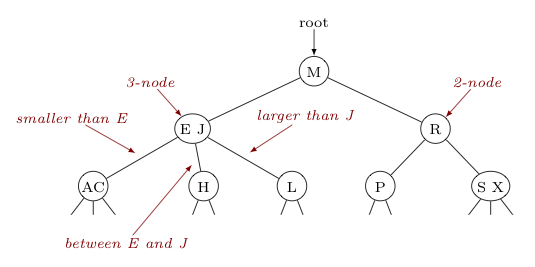The example 2-3 tree above contains two 3-nodes, one containing keys $E,J$ and the other containing keys $S,X$. Looking at the 3-node $EJ$ above, note how the symmetric order requires its left subtree contain only keys smaller than its first key ($E$), its center subtree contain only keys between its two keys ($E$ and $J$), and its right tree contains only keys larger than its second key ($J$). This is similarly required of every 3-node in the tree. For 2-nodes, the symmetric order requires the same behavior as that seen in binary search trees -- left subtrees contain only keys less than the key stored, right subtrees contain only keys greater than the key stored.

Searching through a 2-3 tree is very similar to searching in a binary tree -- just with more comparisons required as we navigate through 3-nodes, to decide which path down to pursue. As an example, consider how we might find a reference to the node containing $H$ in the tree below:Also like searching in a binary tree, when this process terminates in a null link the key for which we are searching is not present in the tree (i.e., a "miss"). As an example, consider searching for $T$ in the tree below.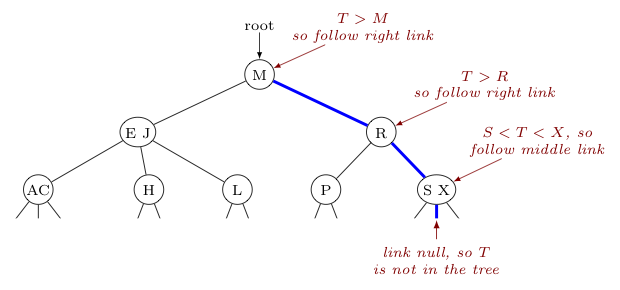Where things get interesting is when one tries to insert a new key-value pair. Remember, a 2-3 tree must be kept both in perfect balance and symmetric order by key. How can we maintain this when adding new things to the tree?

What must be done depends a lot on where the insertion needs to happen.

Inserting into a 2-node leaf is trivial -- we simply convert it to a 3-node leaf and add our new key, as the below two images show.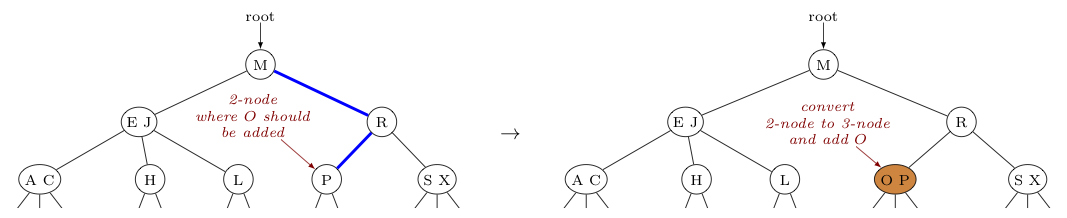Inserting into a 3-node leaf is more complicated. To see how this process works by example, consider the steps to insert the key $D$ into the tree above.

 Step 1:We first navigate down the tree's branches, searching for $D$, the key to add.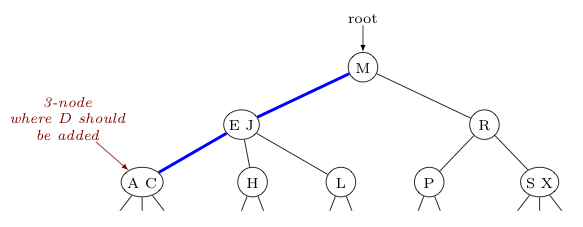Step 2:Now we temporarily convert the 3-node into a 4-node, putting our new key ($D$) into this node (in the correct order, of course).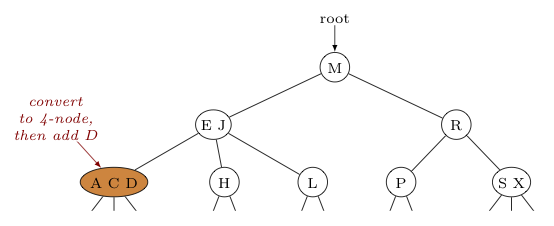Step 3:Then, we split this 4-node into two 2-nodes, inserting the middle key into this node's parent. If this parent is a 2-node, we convert it to a 3-node to accomplish the insertion. If instead the parent is a 3-node, we do the same thing as was just done -- we temporarily convert it to a 4-node, and insert the new key.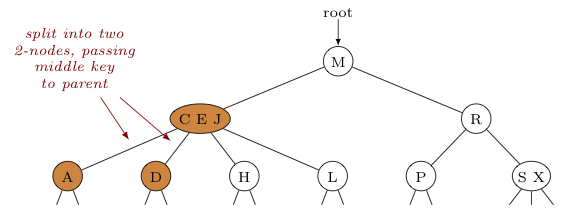Step 4:As long as we have a 4-node, we continue in this way splitting the 4-node into two 2-nodes and passing the middle key to the next parent up the tree. Here, upon splitting the node $CEJ$ here and passing the middle key $E$ to the root node, the process stops. Note how upon completion of the insertion the tree is still perfectly balanced and still in symmetric order!However, it is possible for the root node itself to be turned temporarily into a 4-node, in which case there is one more step. As an example, consider inserting $L$ to the following tree:

 To insert $L$, we first note that $E \lt L \lt S$, and thus navigate to the middle leaf. This is the node where $L$ needs to be inserted.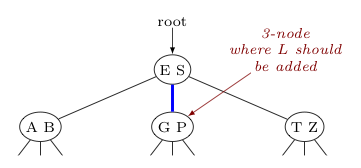We convert this 3-node temporarily into a 4-node, adding $L$ as the key between $G$ and $P$ this time.We split this 4-node into two 2-nodes, passing the middle key $L$ up to the parent.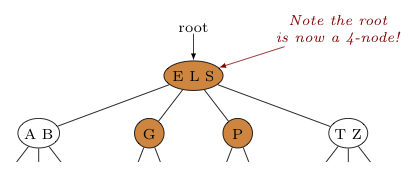Seeing the root is now a 4-node, we split it into three 2-nodes to complete the insertion. This increases the height of the tree by one level, but again keeps the tree perfectly balanced and in symmetric order.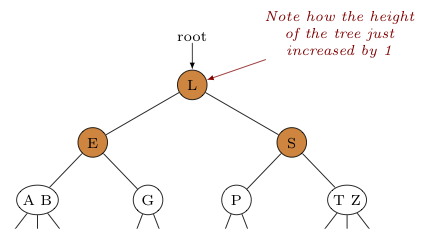In terms of analysis, note that splitting a 4-node only affects its links and the links of its parent. Given the limit on the number of links associated with nodes in a 2-3 tree, this means that the splitting process requires only a constant number of operations, regardless of the size of the tree.

Thus, the cost of insertion will be directly proportional to the height of the tree (i.e., the maximum number of levels where we need to split 4-nodes).

So we naturally then ask ourselves the question: "What is the height of a 2-3 tree with $n$ nodes?"

It should be no surprise that if the 2-3 tree consists of only 2-nodes (i.e., the worst case), the height of the tree is $\log_2 n$. In this situation, things are identical to what we saw in a binary search tree.

In the case where there are only 3-nodes (the best case), the result is similar -- the height is $\log_3 n$. (Can you convince yourself of this?)

More often, the result is somewhere in between.

Either way, the height of the tree -- and consequently, the cost of both searching and insertion -- will be $O(\ln n)$.